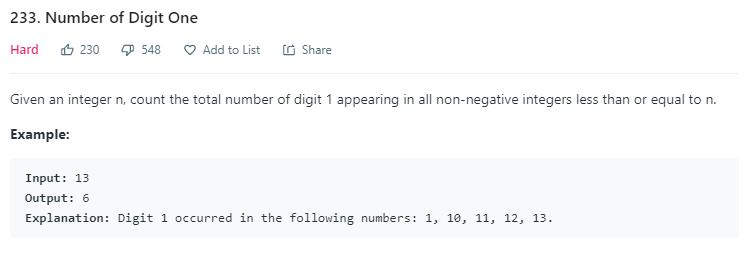# 题目描述（困难难度）# 解法一 暴力

public int countDigitOne(int n) {
int num = 0;
for (int i = 1; i <= n; i++) {
int temp = i;
while (temp > 0) {
if (temp % 10 == 1) {
num++;
}
temp /= 10;
}
}
return num;
}


# 解法二

• d == 0，那么千位上 1 的个数就是 xyz * 1000
• d == 1，那么千位上 1 的个数就是 xyz * 1000 + abc + 1
• d > 1，那么千位上 1 的个数就是 xyz * 1000 + 1000

xyz 固定为上边其中的一个数的时候，abc 可以取 0,1,2...999，也就是 1000 种可能。

d == 1 的时候，千位刚好是 1，此时 abc 可以取的值就是 0abc ，所以多加了 abc + 1

d > 1 的时候，d 如果取 1，那么 abc 就可以取 0999，此时就多加了 1000

如果n = 4560234

xyz 可以取 0 到 455, abc 可以取 0 到 999
4551000 to 4551999 (1000)
4541000 to 4541999 (1000)
4531000 to 4531999 (1000)
...
21000 to   21999 (1000)
11000 to   11999 (1000)
1000 to    1999 (1000)

xyz 可以取 0 到 455, abc 可以取 0 到 999
4551000 to 4551999 (1000)
4541000 to 4541999 (1000)
4531000 to 4531999 (1000)
...
1000 to 1999 (1000)
xyz 还可以取 456, abc 可以取 0 到 234
4561000 to 4561234 (234 + 1)

xyz 可以取 0 到 455, abc 可以取 0 到 999
4551000 to 4551999 (1000)
4541000 to 4541999 (1000)
4531000 to 4531999 (1000)
...
1000 to 1999 (1000)
xyz 还可以取 456, abc 可以取 0 到 999
4561000 to 4561999 (1000)



public int countDigitOne(int n) {
int count = 0;
//依次考虑个位、十位、百位...是 1
//k = 1000, 对应于上边举的例子
for (int k = 1; k <= n; k *= 10) {
// xyzdabc
int abc = n % k;
int xyzd = n / k;
int d = xyzd % 10;
int xyz = xyzd / 10;
count += xyz * k;
if (d > 1) {
count += k;
}
if (d == 1) {
count += abc + 1;
}
//如果不加这句的话，虽然 k 一直乘以 10，但由于溢出的问题
//k 本来要大于 n 的时候，却小于了 n 会再次进入循环
//此时代表最高位是 1 的情况也考虑完成了
if(xyz == 0){
break;
}
}
return count;
}


public int countDigitOne(int n) {
int count = 0;
for (long k = 1; k <= n; k *= 10) {
// xyzdabc
int abc = n % k;
int xyzd = n / k;
int d = xyzd % 10;
int xyz = (xyzd + 8) / 10;
count += xyz * k;
if (d == 1) {
count += abc + 1;
}
}
return count;
}


public int countDigitOne(int n) {
int count = 0;

for (long k = 1; k <= n; k *= 10) {
long r = n / k, m = n % k;
// sum up the count of ones on every place k
count += (r + 8) / 10 * k + (r % 10 == 1 ? m + 1 : 0);
}

return count;
}